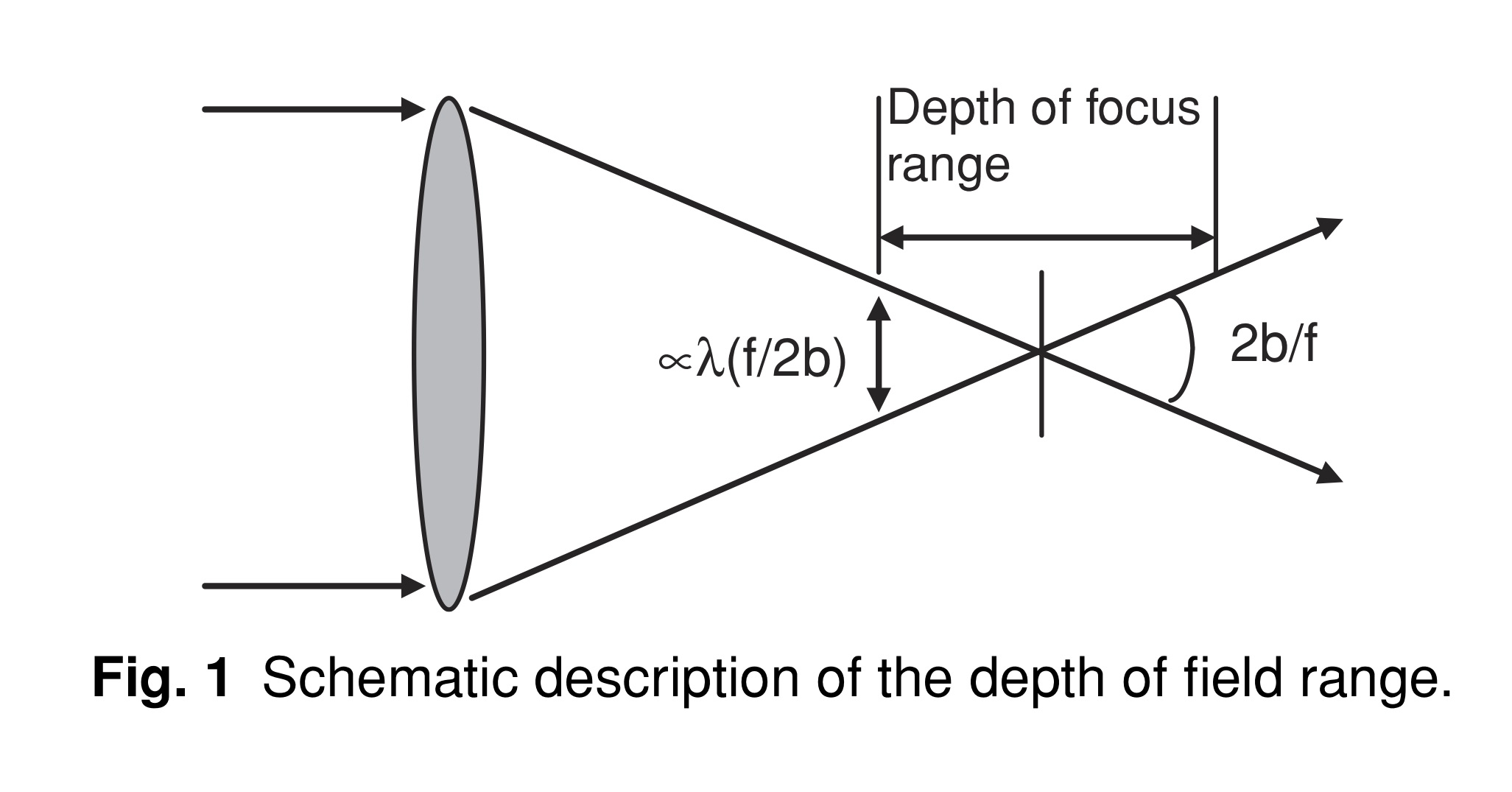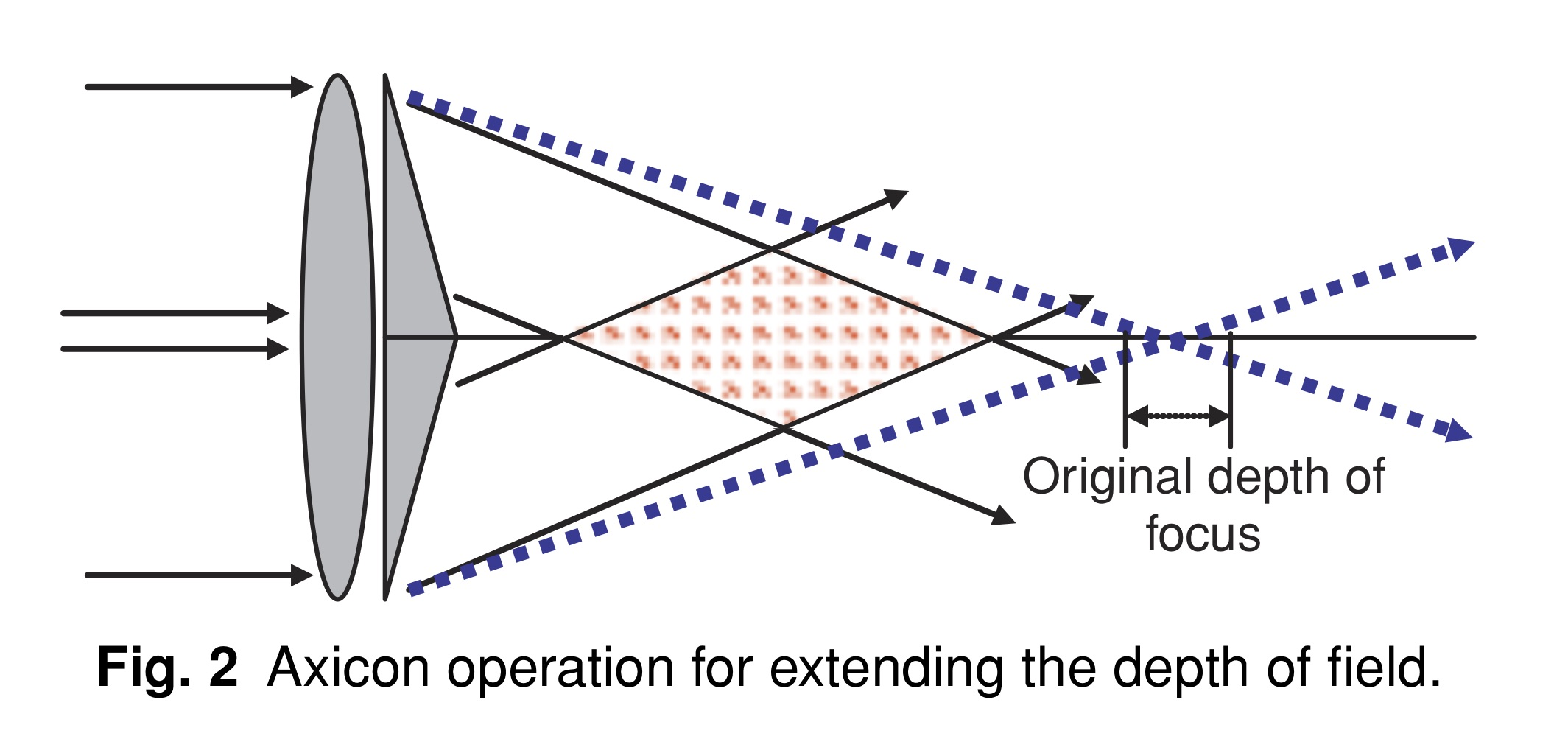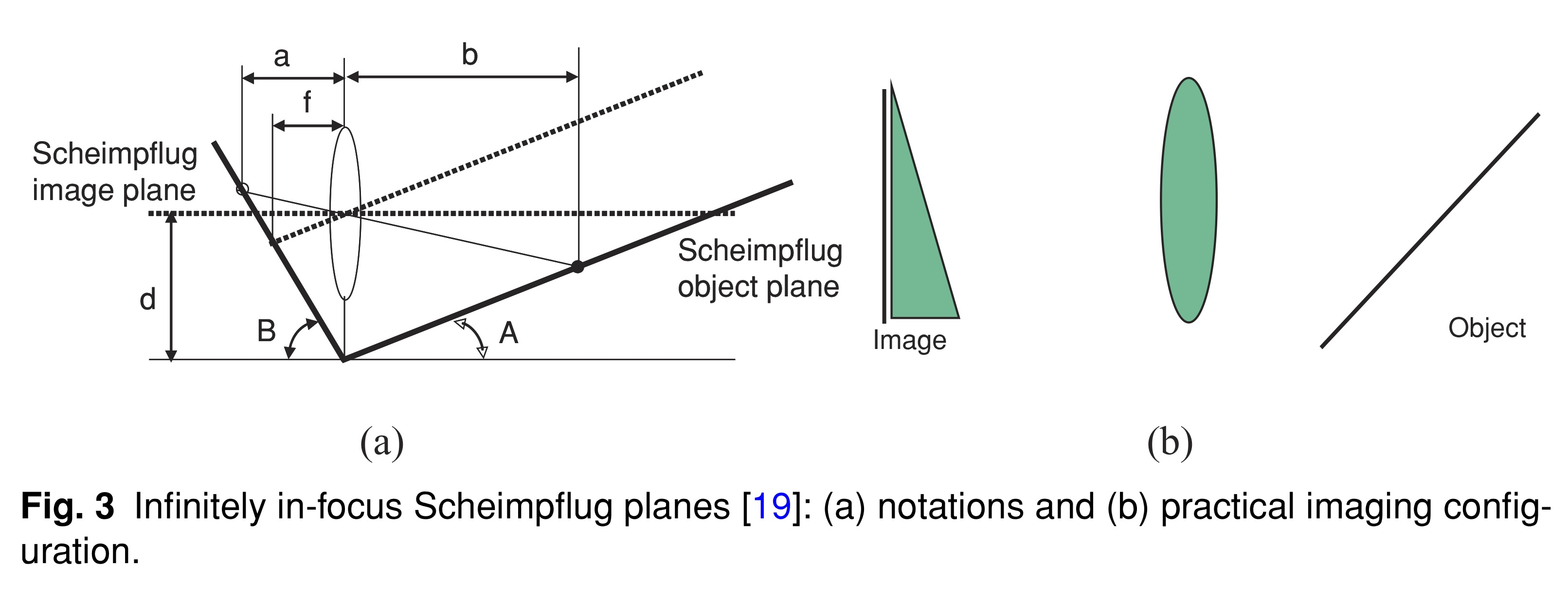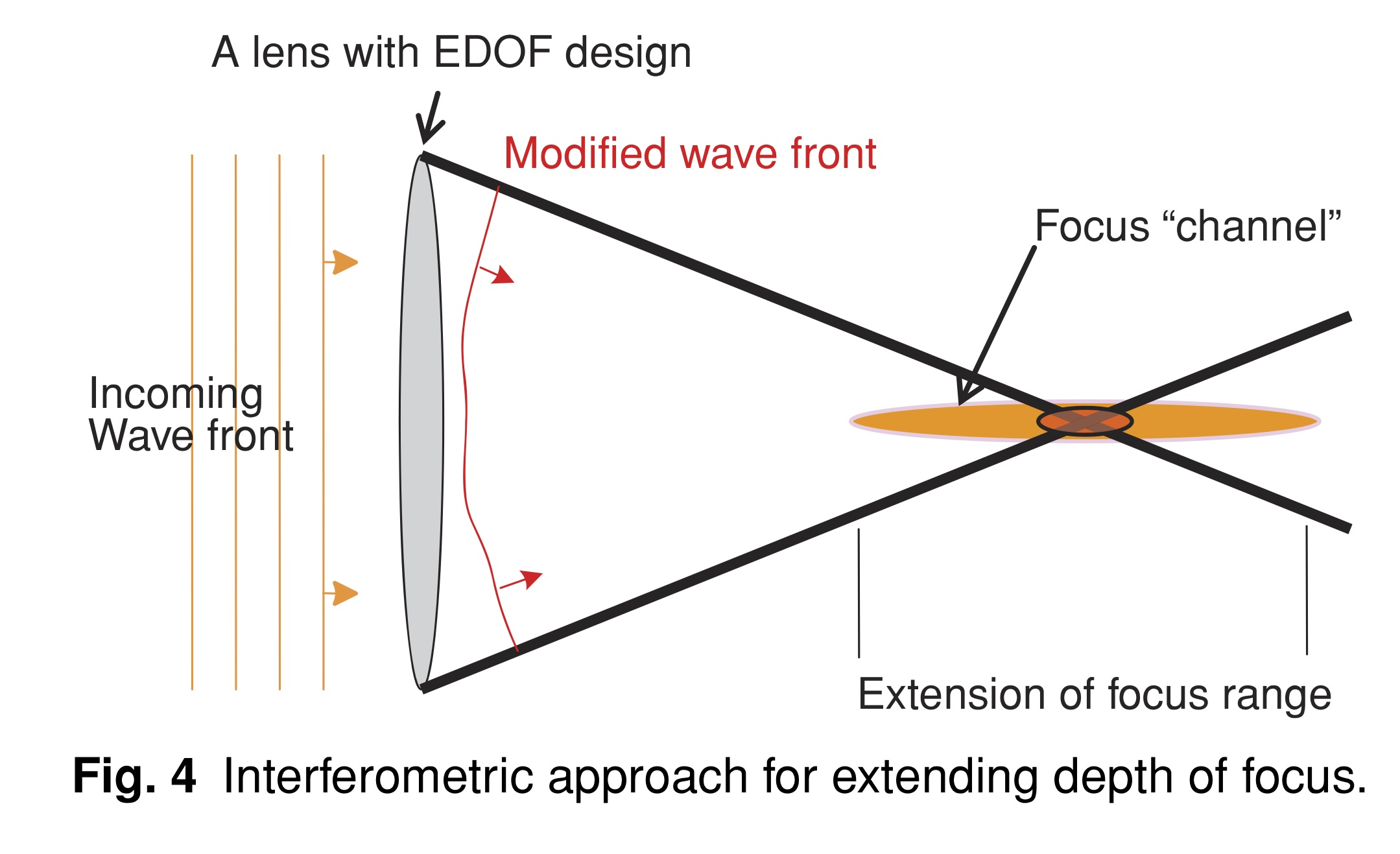# EDoF综述翻译

Zeev Zalevsky "Extended depth of focus imaging: a review," SPIE Reviews 1(1), 018001 (1 January 2010). https://doi.org/10.1117/6.0000001

## Extended depth of focus imaging: a review¶

Zeev Zalevsky

Bar-Ilan University, School of Engineering, Ramat-Gan, 52900, Israel

zalevsz@eng.biu.ac.il

## 1 理论背景¶

$$H\left(\mu_{x}, \mu_{y}\right)=\frac{\int_{-\infty}^{\infty} \int_{-\infty}^{\infty} P\left(x+\frac{\lambda Z_{i} \mu_{x}}{2}, y+\frac{\lambda Z_{i} \mu_{y}}{2}\right) P^{*}\left(x-\frac{\lambda Z_{i} \mu_{x}}{2}, y-\frac{\lambda Z_{i} \mu_{y}}{2}\right) \mathrm{d} x \mathrm{d} y}{\int_{-\infty}^{\infty} \int_{-\infty}^{\infty}|P(x, y)|^{2} \mathrm{d} x \mathrm{d} y} \tag{1}$$

$$H\left(\mu_{x}, \mu_{y}\right)=\frac{\text { Area of overlap }}{\text { Total area }}=\frac{\iint_{A\left(\mu_{x}, \mu_{y}\right)} \mathrm{d} x \mathrm{d} y}{\iint_{A(0,0)} \mathrm{d} x \mathrm{d} y} \tag{2}$$

$$P(x, y)=|P(x, y)| \exp [i k W(x, y)] \tag{3}$$

$$W(x, y)=W_{m} \frac{\left(x^{2}+y^{2}\right)}{b^{2}} \tag{4}$$

$$W_{m}=\frac{\Psi \lambda}{2 \pi} \tag{5}$$

$$\Psi=\frac{\pi b^{2}}{\lambda}\left(\frac{1}{Z_{i}}+\frac{1}{Z_{o}}-\frac{1}{f}\right) \tag{6}$$

$$\frac{1}{Z_{i}}+\frac{1}{Z_{o}}=\frac{1}{f} \tag{7}$$

\begin{aligned} H\left(\mu, W_{m}\right) &=\frac{\int_{-\infty}^{\infty} P\left(x+\frac{\lambda Z_{i} \mu}{2}\right) P^{*}\left(x-\frac{\lambda Z_{i} \mu}{2}\right) \mathrm{d} x}{\int_{-\infty}^{\infty}|P(x)|^{2} \mathrm{d} x} \\ &=\frac{\int_{A(\mu)} \exp \left[\frac{i k W_{m} \lambda Z_{i} \mu x}{b^{2}}\right] \mathrm{d} x}{2 b} \end{aligned} \tag{8}

$$H(\mu)=\left(1-\frac{|\mu|}{2 \mu_{\mathrm{c.o.}}}\right) \operatorname{sinc}\left\{\frac{8 W_{m} \pi}{\lambda}\left(\frac{\mu}{2 \mu_{\mathrm{c.o.}}}\right)\left(1-\frac{\mu}{2 \mu_{\mathrm{c.o.}}}\right)\right\} \tag{9}$$

\begin{aligned} H(\mu) & \approx \frac{\int_{A(0)} \exp \left[\frac{i k W_{m} 2 \lambda Z_{i} \mu x}{b^{2}}\right] \mathrm{d} x}{2 b} \\ &=\frac{\int_{-b}^{b} \exp \left[\frac{4 \pi i W_{m} Z_{i} \mu x}{b^{2}}\right] \mathrm{d} x}{2 b} \\ &=\frac{1}{2 b} \cdot \int_{-\infty}^{\infty} \operatorname{rect}\left(\frac{x}{2 b}\right) \exp \left[\frac{4 \pi i W_{m} Z_{i} \mu x}{b^{2}}\right] \mathrm{d} x \\ &=\operatorname{sinc}\left(\frac{4 W_{m} \pi \mu Z_{i}}{b}\right) \end{aligned} \tag{10}## 2 概述：扩展景深的方法¶$$\frac{1}{a}+\frac{1}{b}=\frac{1}{d}(\tan B+\tan A)=\frac{1}{f} \tag{11}$$

Scheimpflug成像中，图3(a)中指定的线上物和像始终对焦清晰，因此沿这些平面定位的物体将始终成像于焦点上。这种方法的主要缺点是，在“渐进”镜片的情况下，视野非常有限，因为每个轴向距离都在不同的侧位成像。$$\Delta_{B}=\frac{\Delta}{\left(1-\frac{n_{0}}{n_{\mathrm{e}}}\right)} \tag{12}$$

## 3 老视与人类视觉¶

【这一部分看起来比较陈旧，翻译略】，提到可能可以用EDoF来阻止儿童近视进展。

## 参考文献¶

In [ ]: